This article, "Depreciation Expense: Straight-Line Method Explained with a Finance Lease Example and Journal Entries," originally appeared on LeaseQuery.com.

Summary provided by MaterialAccounting.com: This article defines depreciation expense and uses examples to explain the methods available for calculating it.

## What is depreciation?

Depreciation is a way to account for the reduction of an asset’s value as a result of using the asset over time. Depreciation generally applies to an entity’s owned fixed assets or to its right-of-use (ROU) assets arising from finance leases for lessees.

Depreciation expense allocates the cost of a company’s use of an asset over its expected useful life. The expense is an income statement line item recognized throughout the life of the asset as a “non-cash” expense.

Accumulated depreciation is the associated balance sheet line item for depreciation expense. Depreciation expense is recorded as a debit to expense and a credit to a contra-asset account, accumulated depreciation. The contra-asset account is a representation of the reduction of the fixed asset’s value over time. The accumulated depreciation account has a normal credit balance, as it offsets the fixed asset, and each time depreciation expense is recognized, accumulated depreciation is increased. An asset’s net book value is its cost less its accumulated depreciation.

Accumulated depreciation is carried forward on the balance sheet until the related asset is disposed of and reflects the total reduction in value of the asset over time, or in other words, the total amount of depreciation expense recorded in previous periods.

## Depreciation methods

Under US GAAP, there are four methods of calculating depreciation a company may use:

1. Straight-line method of depreciation
2. Double-declining balance method
3. Sum-of-the-years’-digits depreciation method
4. Units of production depreciation method

Below we will describe each method and provide the formula used to calculate the periodic depreciation expense.

### Straight-line method of depreciation

The straight-line method of depreciation is the most common method used to calculate depreciation expense. It is the simplest method because it equally distributes the depreciation expense over the life of the asset.

The only inputs required to calculate depreciation expense using the straight-line depreciation method are:

• The cost of the asset: the amount the business paid for the asset or for the use of the asset
• The salvage value: sometimes called scrap value or residual value, is the estimate of the amount the company expects to receive for the asset if sold at the end of its useful life
• The useful life of the asset: the estimated amount of time the asset is expected to be functionally used before it needs to be replaced or disposed

Below we have provided the formula to calculate straight-line depreciation expense: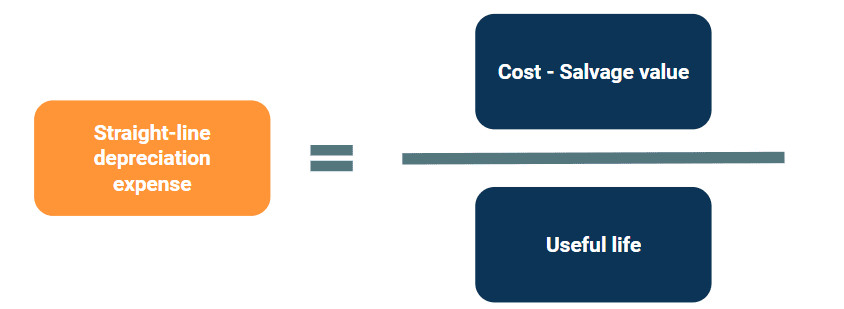Because this method is the most universally used, we will present a full example of how to account for straight-line depreciation expense on a finance lease later in our article.

### Alternative depreciation methods

While the straight-line depreciation method is typically used, other methods of depreciation are acceptable for businesses to use under US GAAP to calculate depreciation expense.

#### Double-declining balance method

The double-declining balance method of depreciation does not recognize depreciation expense evenly over the life of the asset, but rather, takes into account that assets are generally more productive the newer they are, and become less productive in their later years. Because of this, the double-declining balance depreciation method records higher depreciation expense in the beginning years and less depreciation in later years. This method is commonly used by companies with assets that lose their value or become obsolete more quickly.

To calculate depreciation using this method, a rate of depreciation is calculated and multiplied by the book value each year. The formula to calculate depreciation expense using this method is as follows: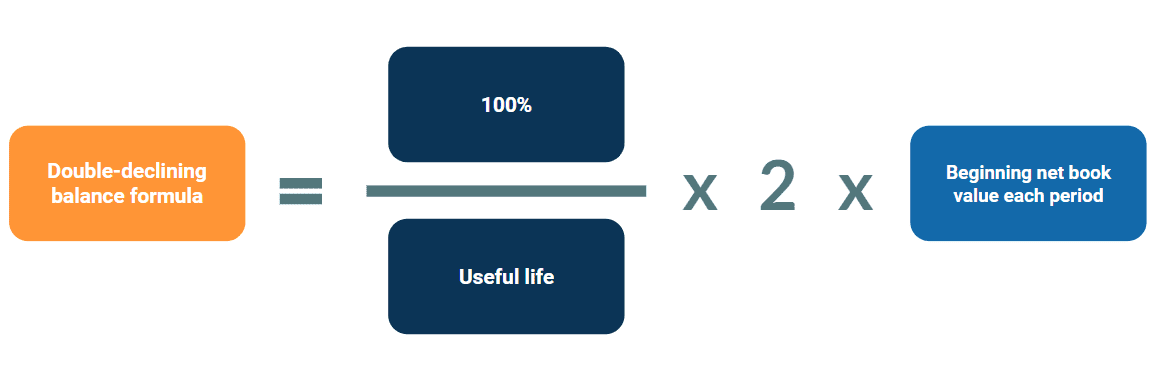The term “double-declining balance” is due to this method depreciating an asset twice as fast as the straight-line method of depreciation. The “2” in the formula represents the acceleration of deprecation to twice the straight-line depreciation amount. However, when using the double-declining balance method of depreciation, an entity is not required to only accelerate depreciation by two. They are able to choose an acceleration factor appropriate for their specific situation.

#### Sum-of-the-years’-digits depreciation method

Similarly to the double-declining balance method, the sum-of-the -years’-digits method accelerates depreciation, resulting in higher depreciation expense in the earlier years of an asset’s life and less in later years.

This method is calculated by adding up the years in the useful life and using that sum to calculate a percentage of the remaining life of the asset. The percentage is then applied to the cost less salvage value, or depreciable base, to calculate depreciation expense for the period.

For example, if an asset has a useful life of 5 years, summing the digits of the five years of the asset’s useful life looks like this: 1 + 2 + 3 + 4 + 5 = 15. Each year, the depreciable base is multiplied by the percentage of the remaining useful life to determine the annual depreciation expense. The annual expense is then allocated evenly over the year in each financial period.

For further clarity, for an asset with a 5-year useful life, the annual depreciation expense for the first year would be one third (⅓ = 5 / 15) of the asset’s value. In year two the annual depreciation expense percentage would be 4/15 and in year three the percentage would be one fifth (⅕ = 3/15), and so on.

#### Units of production depreciation method

Unlike the other methods, the units of production depreciation method does not depreciate the asset solely based on time passed, but on the units the asset produced throughout the period.

This method first requires the business to estimate the total units of production the asset will provide over its useful life. Then a depreciation amount per unit is calculated by dividing the cost of the asset minus its salvage value over the total expected units the asset will produce. Each period the depreciation per unit rate is multiplied by the actual units produced to calculate the depreciation expense.

Below are the two formulas used in the units of production depreciation method: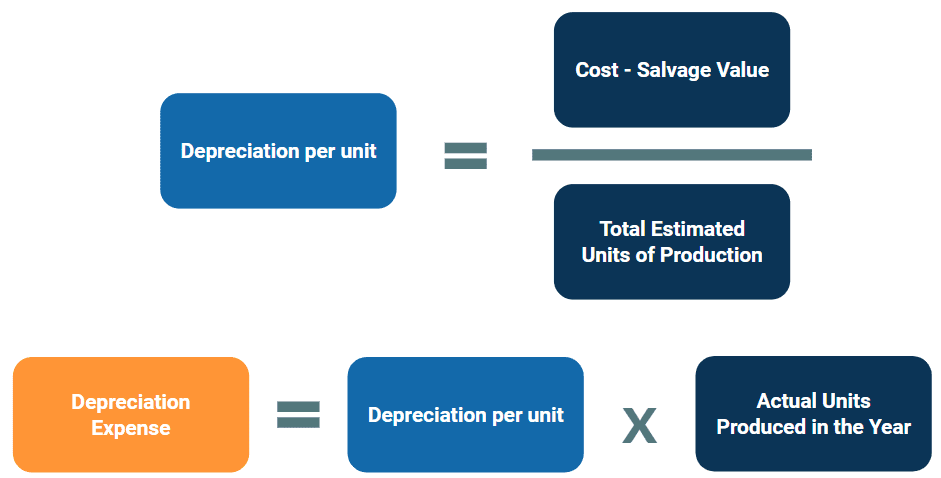Regardless of the depreciation method used, the total depreciation expense (and accumulated depreciation) recognized over the life of any asset will be equal. However, the rate at which the depreciation is recognized over the life of the asset is dictated by the depreciation method chosen.

## Example: Straight-line depreciation with a finance lease

Now, let’s consider a full example of a finance lease to illustrate straight-line depreciation expense.

Reed, Inc. leases equipment for annual payments of \$100,000 over a 10 year lease term. Payments are due in advance on January 1 of each year.

This lease qualifies as a finance lease because it is written in the agreement that ownership of the equipment automatically transfers to Reed, Inc. (the lessee) when the lease terminates. To evaluate the lease classification, we used the capital vs. operating lease criteria test.

• Payments: \$100,000 annually in advance
• Salvage value: \$0
• Lease term: 10 years
• Lease start date: 1/1/2022
• Lease end date: 12/31/2031
• Useful life of equipment: 10 years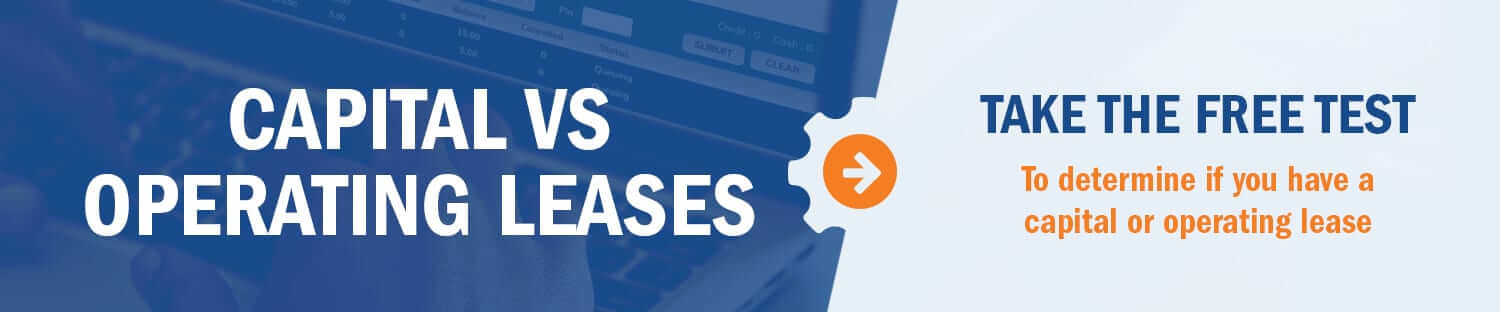Reed, Inc. also evaluates the incremental borrowing rate for the lease to be 4%. For this example we will assume no other lease incentives, accruals, or initial direct costs are applicable for this lease.

Using the facts and circumstances presented, we can use LeaseQuery’s present value calculator to calculate the present value of the lease payments. This is the value we will record for the ROU asset and what will be depreciated. In order to do so, input annual payments of \$100,000, a 10 year lease term, and a 4% discount rate. At commencement, the lessee records a lease asset and lease liability of \$843,533.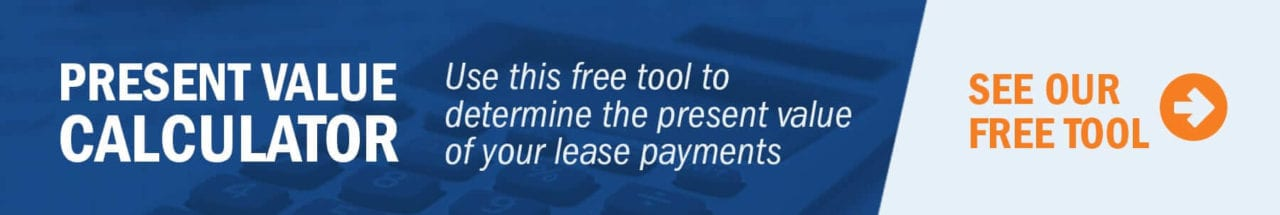### Initial journal entries

To recognize the ROU asset and lease liability at lease commencement, the lessee makes the following entry:### Straight-line depreciation expense calculation

In our explanation of how to calculate straight-line depreciation expense above, we said the calculation was (cost – salvage value) / useful life. For a finance lease, it is important to distinguish whether the agreement is a “strong form” or a “weak form” finance lease to determine whether the useful life or the lease term is used as the depreciable base term.

A strong form finance lease is one that has a transfer of ownership, a bargain purchase option (BPO), or a purchase option the lessee is reasonably certain to exercise. With a strong form lease, the asset is depreciated over the useful life of the asset as it is assumed the lessee will own the asset at the end of the lease term. For weak form finance leases where the lessor retains ownership of the asset at the end of the lease term, the asset is depreciated over the shorter of the useful life or the lease term.

In our example, the title transfers, which means at the end of the lease term the lessee will own the asset and continue depreciating it. However, the useful life of the equipment in this example equals the lease term (10 years) so at the end of the lease, the asset will be depreciated to \$0.

To calculate the straight-line depreciation expense, the lessee takes the gross asset value calculated above of \$843,533 divided by 10 years to calculate an annual depreciation expense of \$84,353.

Below is the amortization schedule for both the ROU asset and the lease liability: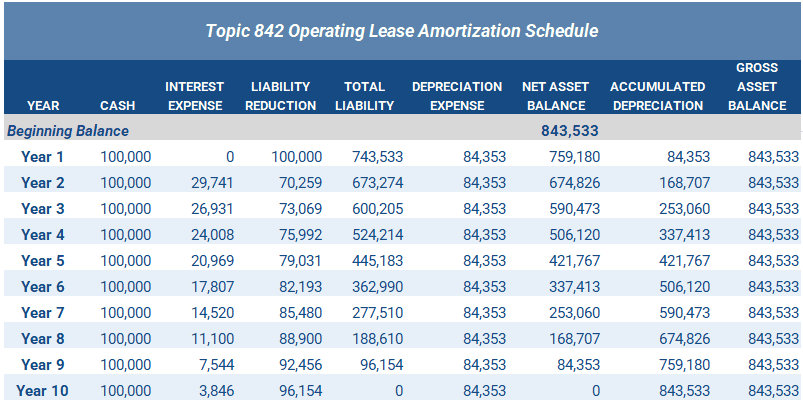### Annual journal entries

Throughout the year, the lessee recognizes straight-line depreciation evenly per month as a reduction in the asset value recorded to the depreciation expense account and accumulated depreciation. The lessee makes the following entry in Year 1 to recognize the \$100,000 payment and annual depreciation of \$84,353:In subsequent entries, the lessee will make journal entries to recognize the depreciation expense on the asset, the reduction to the lease liability’s principal balance, and interest expense accrual on the outstanding liability. For example, in year 2, the lessee makes the following entry: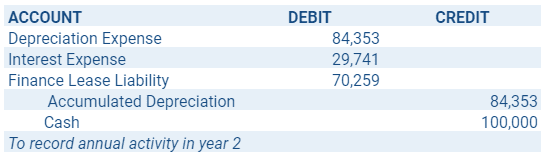As you can see from the amortization table, this continues until the end of Year 10, at which point the total asset and liability balances are \$0. Further, the full value of the asset resides in the accumulated depreciation account as a credit. Combining the two amounts equals a net book value of \$0.

## Summary

Depreciation expense is the recognition of the reduction of value of an asset over its useful life. Multiple methods of accounting for depreciation expense exist, but the straight-line method is the most commonly used. In this article, we covered the different methods used to calculate depreciation expense, and went through a specific example of a finance lease with straight-line depreciation expense.

The calculations required to create an amortization schedule for a finance lease can be complex to manage and track within Excel. A software solution such as LeaseQuery can assist in the calculation and management of depreciation expense on your finance leases.